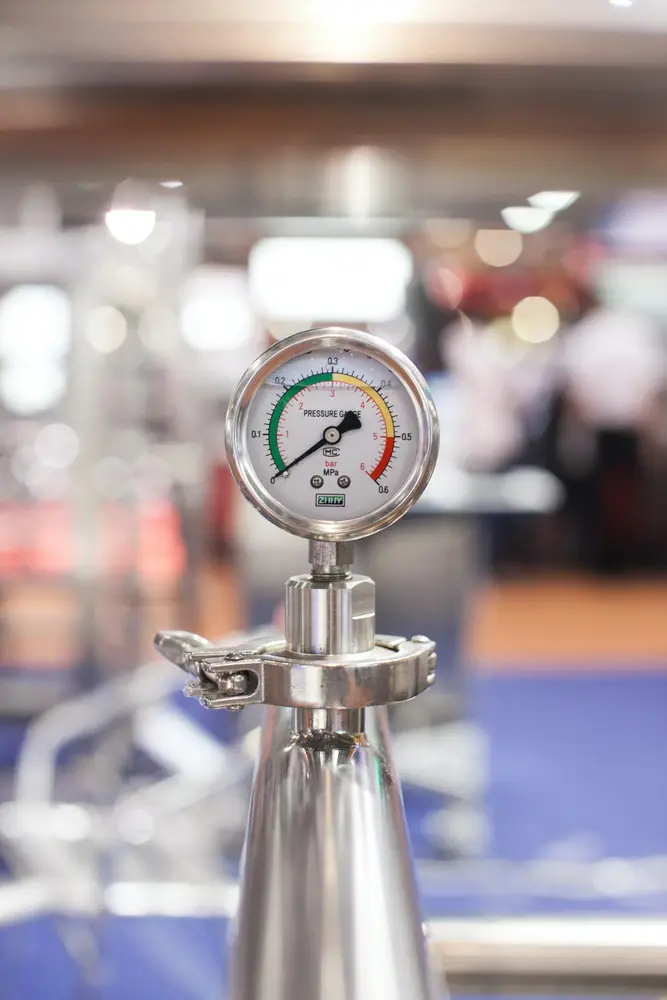# Forumulae

Here are the formulae for speed, velocity and acceleration. You will probably be expected to learn these for your GCSE exam:

• Average speed = distance moved / time taken
• Average velocity = distance moved in a certain direction / time taken
• Acceleration = change in velocity / time taken
• Remember all of these formulae can be rearanged.
• e.g. distance = average speed x time taken
• Below are the formulae you can use when calculating problems which have uniform acceleration (constant acceleration). You probably will be only expected to be able to use these and not learn them but make sure you check this with your teacher.
• v = u + at
• s = (u + v)/2 x t
• s = ut + ½at2
• v2 = u2 + 2as
• What the letters stand for:
• u = initial velocity
• t = time
• v = final velocity
• s = distance travelled
• a = acceleration (must be constant)
Forces and motion

#### Acceleration

##### Acceleration

Forces and motion

#### Forces

##### Forces

Forces and motion

#### Hooke's Law

##### Hooke's Law

Forces and motion

#### Momentum

##### Momentum

Forces and motion

#### Pressure

##### Pressure

Forces and motion